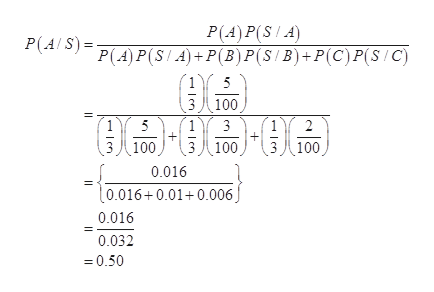# A beverage plant has three lines (line A, line B and line C) for bottling the beverages. Line A bottles at a rate of 800 per hour, of which 5 percent have short fill; Line B bottles at a rate of 700 per hour, of which 3 percent have short fill; Line C bottles at a rate of 500 per hour, of which 2 percent have short fill. The lines operate for the same number of hours per day. A bottle selected at random from the production is found to have short fill. What are the probabilities that this bottle comes from line A, line B, or line C?

Question
110 views

A beverage plant has three lines (line A, line B and line C) for bottling the beverages. Line A bottles at a rate of 800 per hour, of which 5 percent have short fill; Line B bottles at a rate of 700 per hour, of which 3 percent have short fill; Line C bottles at a rate of 500 per hour, of which 2 percent have short fill. The lines operate for the same number of hours per day. A bottle selected at random from the production is found to have short fill. What are the probabilities that this bottle comes from line A, line B, or line C?

check_circle

Step 1

Let A be the event of selecting line A, B be the event of sleeting line B and C be the event of selecting line C.

Since there are three lines in a beverage plant, hence the probability of selecting any line is equally likely and mutually exclusive.

Step 2

It is given that the line A has 5 percent short fill bottles, line B has 3 percent short fill bottles and Line C has 2 percent short fill bottles.

Let S be the event that the selected bottle is short fill, then the priory probability are,

Step 3

The probability that the short fill bo...help_outlineImage TranscriptioncloseP(A) P(S/A) P(A) P(SA)+P(B) P(S B)+P(C) P(S/C) P(A S) 5 3八100 5 2 3八100 100 100 0.016 t0.016+0.01+0.006J 0.016 0.032 =0.50 fullscreen

### Want to see the full answer?

See Solution

#### Want to see this answer and more?

Solutions are written by subject experts who are available 24/7. Questions are typically answered within 1 hour.*

See Solution
*Response times may vary by subject and question.
Tagged in

### Probability